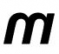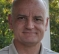## OFDM samplingStarted by 6 years ago13 replieslatest reply 6 years ago3809 views

In OFDM system, The relation N=BW/Δf, where is number of subcarriers, BW is the available bandwidth and Δ is the subcarrier spacing (subcarrier bandwidth) is reasonable for me.

I don't understand how the discrete data that entered to IFFT is sampled, and why I need to perform sampling for this type of data (it is already discrete) , and what is the relation between the sampling frequency value and the number of subcarriers?

[ - ]Simply, you may think that for period of one symbol time domain signal is not defined through samples. Instead, the signal is defined in frequency domain, then through IFFT one translates frequency domain data to time domain samples. Now from discrete frequency domain you get still discrete, but time domain samples. The nature of complex (I)FFT is such that you consume N frequency domain complex magnitudes to produce same N time domain complex samples, which are fed to quadrature transmitter. Perhaps, the misconception is frequency domain data should not be treated as samples. Rather, the whole block of frequency domain data allows to produce number of time domain samples.

[ - ]Thanks sir, your wards "Now from discrete frequency domain you get still discrete, but time domain samples" that means the time and frequency domain signals are discrete? and if the sampling frequncy larger than the availble bandwidth, and the subcarrier spacing constant, in this case number of subcarriers increase. I don't catch how the number of subcarriers N increase, in spite of the available bandwidth BW and the subcarrier space ΔfN=BW/Δf are constant, and , as i know N=BW/ΔfN=BW/Δf

[ - ]Sometimes the confusion is related to the fact that some/many of the frequency domain bins may not be occupied for the inverse FFT.

In WiFi for example, only 52 bins are used, 48 of which are modulated and 4 of which are pilot carriers.  If we use a 64 point IFFT (most often chosen as a 2^M length) to create the time domain data, then the sampling rate must be 64 times the sub-carrier spacing.

In the following picture the distinction is made between the BW and OBW, where OBW means "occupied band-width".  This may be where the most confusion occurs.I have never seen a case where success was achieved with all the sub-carriers occupied.

As a further elaboration, if we choose to have a 128 point IFFT, then there are still only 52 subcarriers active, but now our time domain sampling rate will be 40 MHz.  As @rrlagic has stated, the sampling rate is defined by the length of the FFT multiplied by the sub-carrier spacing.

I hope that his is helpful.

David

[ - ]Thanks David for your help, the example i work on the number of subcarriers 512 (power 2 number), bandwidth BW 2560 Hz and the frequency spacing 5Hz,the auther used 192KHz sampling frequency (much longer than BW), and so on the number of subcarriers increased (pad the added subcarriers). Is that means the bandwidth increased, or the new number of subcarriers are sent through the same bandwidth?

[ - ]The connection of sampling rate(Fs) is that it physically decides the bw as follows:

signal bw = Fs/nFFT * Number of active carriers.

where Fs/nFFT = bw of one bin

For example lte 5MHz is obtained from an nFFT of 512 points, sampled @ 7.68MHz. In lte 5 there are 300 carriers so:

lte5 bw = 7.68/512 * 300 = 4.5 MHz (nominally 5MHz due to added guard spacing)

You can draw similar conclusions in your case but your figures are not that clear to me.

The choice of sampling rate is to do with obtaining the right carrier spacing which in the case of lte5 = 7.68/512 = 15KHz

[ - ]Thanks Kaz for your simple explaination, another question please, the actual bandwidth required to transmit nFFT is fs (7.68MHz) or signal bandwidth (5MHz)?

[ - ]The actual bw in tx signal is 5MHz.

Fs is only resident of digital domain and is lost in space. The signal inside digital domain in fact will be upsampled to whatever the designer chooses for final DAC and total bw required for number of such lte signals. This wouldn't and shouldn't change the bw of 5MHz at all. I have upsampled to 360MHz or 245 depending on system.

[ - ]"Fs is only resident of digital domain and is lost in space" what do you mean with lost in space?

What is the defects of increasing sampling frequency (Fs>>BW)?

It would be nice to help me with a good source in this topic.

[ - ]"Fs is only resident of digital domain and is lost in space"  That is meant to be metaphor. as Fs is applied through a digital clock that ends at DAC. What goes out is the signal.

The receiver has then the headache to recover clock and possibly frequency centre.

Fs minimum for digital processing is based on sampling theorem but at DAC most systems go much much higher to ease RF filter design.

When you increase Fs you should upsample the signal to new sampling rate so that bw is not changed, i.e. you create new samples in between original samples such that the signal stays nice and correct but just had more samples per unit time.

[ - ][ - ]I believe that you have all the information (and more) that you need.

If the sampling rate that the author was using is 192kHz, and the sub-carrier frequency is 5 Hz, then you need 192kHz/5Hz = 38400 length DFT to get each sub-carrier to land exactly in one bin. Only 512 of the bins will be non-zero.

I think that it is that simple for you.

Just a word of caution related to the FFT length.  If you used a 512 point FFT with 2560 sampling rate, then you have to have EXACTLY the right sampling rate, sampling phase, main carrier frequency and main carrier phase to decode the data.

Good luck.

David

[ - ]Hey,

Hang on. Let me explain something to you which you might not find directly in any of the text book until you do some hands on.

Sampling rate in 'C' or Matlab have no meaning until you place the samples one by one to DAC and decide the sending rate of each sample. Which means that "inverse of" time taken between sending one IQ sample to DAC to next IQ sample will decide the real sampling rate in Hz or MHz.

If you don't beleive me just generate a plot with 10 sinusoidal samples in one period in matlab and plot it. What is the sampling frequency here?

If you right 0 second for first sample on x-axis and 1 sec for last sample then it becomes 0.1 Hz but if you write 100 sec on x-axis for 10th sample then fs becomes 0.01 Hz.

See you haven't changed anything but fs has changed depends on how samples are placed to one another in time domain.

Once decided in time domain, you can use fs in freuency domain and each fft sample will fs/N apart.

Establishing that if you are clear in time domain, you are sorted  about fs now we take your numbers.

You example would have been simple if sampling rate is 2 to the power multiple of 2.56 kHz bandwidth so that IFFT could still be used.

If target is to use exactly 5 Hz spaced carrier then sampling frequency can not be 192 kHz (while still using IFFT/FFT instead of IDFT/DFT).This is because fs chosen by you is 75  times you bandwidth required. 512 point IFFT for 5Hz space 2.56 kHz bandwidth is fine but there isno IFFT defined for 38400 samples.

If you have IDFT in mind then you can still do it by leaving modulating every 75 the carrier to your required data before IDFT and leaving rest of the bins at 0 value.

With 192kHz sampling but 512 samples only, you get 375 Hz spaced carrier and not 5 Hz spaced. To achieve 5 Hz spacing you need at least 38400 bins and if you want to use fft, nearest 2 power multiple is 65536 carrier.

So if you want to  use this much high sampling rate knowing that acutal bandwidth requirement is 2.56 kHz what you can do here is that use 65536 carrier with 192 kHzsampling. This will give you 2.92 Hz spaced carriers. Use required number of middle carriers which makes total carrier bandwidth to 2.56 kHz. fill 0's for restof the carrier and then take IFFT.

Hope this clarify few things...

[ - ]